kirbystep4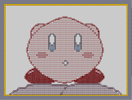Hover over the thumbnail for a full-size version.

Author funman author:funman n-art rated 2006-09-23 4 by 16 people. \$kirbystep4#funman#none#00000000000000000000000000000000000000000000000000000000000000000000000000000000000000000000000000000000000000000000000000000000000000000000000000000000000000000000000000000000000000000000000000000000000000000000000000000000000000000000000000000000000000000000000000000000000000000000000000000000000000000000000000000000000000000000000000000000000000000000000000000000000000000000000000000000000000000000000000000000000000000000000000000000000000000000000000000000000000000000000000000000000000000000000000000000000000000000000000000000000000000000000000000000000000000000000000000000000000000000000000000000000000000000000000000000000000000000000000000000000000000000000000000000000000000000000000000000000000000|12^438,486!12^444,486!12^450,480!12^456,480!12^462,474!12^468,474!12^474,468!12^480,468!12^486,462!12^492,462!12^498,456!12^504,456!12^510,450!12^516,450!12^522,444!12^528,444!12^588,384!12^594,378!12^594,372!12^600,366!12^600,360!12^606,354!12^606,348!12^606,342!12^612,336!12^612,330!12^612,324!12^618,312!12^618,306!12^618,300!12^618,288!12^618,294!12^612,318!12^432,486!12^426,492!12^420,492!12^414,492!12^408,492!12^396,498!12^390,498!12^378,498!12^384,498!12^612,282!12^612,276!12^612,270!12^612,264!12^606,258!12^606,252!12^606,246!12^600,240!12^600,234!12^594,228!12^594,222!12^534,438!12^540,432!12^546,426!12^558,414!12^552,420!12^564,408!12^576,396!12^570,402!12^582,390!12^588,216!12^582,210!12^576,204!12^570,198!12^564,192!12^558,186!12^552,180!12^546,174!12^540,168!12^534,162!12^528,156!12^528,156!12^528,156!12^522,156!12^516,150!12^510,150!12^504,144!12^498,144!12^492,138!12^486,138!12^480,132!12^474,132!12^462,126!12^468,126!12^456,120!12^450,120!12^444,114!12^438,114!12^432,114!12^426,108!12^420,108!12^414,108!12^408,108!12^372,108!12^366,108!12^360,108!12^354,108!12^402,102!12^396,102!12^390,102!12^384,102!12^378,102!12^348,114!12^336,114!12^342,114!12^330,120!12^324,120!12^318,126!12^312,126!12^306,132!12^300,132!12^294,138!12^288,138!12^282,144!12^276,144!12^270,150!12^264,150!12^258,156!12^252,156!12^246,162!12^240,168!12^240,168!12^234,174!12^228,180!12^222,186!12^216,192!12^210,198!12^204,204!12^198,210!12^192,216!12^186,222!12^186,228!12^180,234!12^174,246!12^174,258!12^174,252!12^168,264!12^168,270!12^168,276!12^168,282!12^162,288!12^162,294!12^162,300!12^162,306!12^162,312!12^168,318!12^168,330!12^168,324!12^168,336!12^174,342!12^174,348!12^174,354!12^180,360!12^180,366!12^186,372!12^186,378!12^192,384!12^198,390!12^204,396!12^210,402!12^216,408!12^222,414!12^228,420!12^234,426!12^240,432!12^246,438!12^252,444!12^258,450!12^264,450!12^270,456!12^276,456!12^282,462!12^288,462!12^294,468!12^300,468!12^306,474!12^312,474!12^318,480!12^324,480!12^336,486!12^342,486!12^348,492!12^354,492!12^366,492!12^360,492!12^402,492!12^372,492!12^420,120!12^408,120!12^396,120!12^384,120!12^372,120!12^360,120!12^396,108!12^384,108!12^444,120!12^444,132!12^432,120!12^432,132!12^420,132!12^408,132!12^396,132!12^384,132!12^372,132!12^360,132!12^348,132!12^348,120!12^336,120!12^336,132!12^324,132!12^312,144!12^324,144!12^336,144!12^336,144!12^348,144!12^360,144!12^360,144!12^372,144!12^384,144!12^384,144!12^396,144!12^408,144!12^432,144!12^456,144!12^420,144!12^456,132!12^468,132!12^480,144!12^468,144!12^444,144!12^492,156!12^480,156!12^468,156!12^444,156!12^456,156!12^432,156!12^420,156!12^408,156!12^396,156!12^384,156!12^372,156!12^360,156!12^348,156!12^336,156!12^324,156!12^312,156!12^300,156!12^300,144!12^288,156!12^276,156!12^252,168!12^264,168!12^276,168!12^288,168!12^300,168!12^312,168!12^264,156!12^324,168!12^336,168!12^348,168!12^360,168!12^372,168!12^384,168!12^396,168!12^408,168!12^420,168!12^432,168!12^444,168!12^456,168!12^468,168!12^480,168!12^492,168!12^516,168!12^504,168!12^528,168!12^504,156!12^516,156!12^540,180!12^528,180!12^516,180!12^504,180!12^492,180!12^480,180!12^468,180!12^456,180!12^444,180!12^432,180!12^420,180!12^408,180!12^396,180!12^384,180!12^372,180!12^360,180!12^348,180!12^336,180!12^324,180!12^312,180!12^300,180!12^288,180!12^276,180!12^264,180!12^252,180!12^240,180!12^228,192!12^240,192!12^252,192!12^264,192!12^276,192!12^288,192!12^300,192!12^312,192!12^324,192!12^336,192!12^348,192!12^360,192!12^372,192!12^384,192!12^396,192!12^408,192!12^420,192!12^432,192!12^444,192!12^456,192!12^468,192!12^492,192!12^480,192!12^504,192!12^516,192!12^540,192!12^528,192!12^552,192!12^216,204!12^228,204!12^240,204!12^252,204!12^264,204!12^288,204!12^276,204!12^300,204!12^336,204!12^324,204!12^348,204!12^360,204!12^360,204!12^372,204!12^384,204!12^396,204!12^408,204!12^420,204!12^432,204!12^444,204!12^456,204!12^480,204!12^492,204!12^504,204!12^516,204!12^528,204!12^540,204!12^552,204!12^564,204!12^576,216!12^564,216!12^552,216!12^528,216!12^540,216!12^516,216!12^504,216!12^492,216!12^432,216!12^444,216!12^420,216!12^408,216!12^396,216!12^384,216!12^372,216!12^372,216!12^360,216!12^456,492!12^450,492!12^462,492!12^468,498!12^480,498!12^474,498!12^492,498!12^498,498!12^486,498!12^330,486!12^324,492!12^312,492!12^318,492!12^306,498!12^300,498!12^288,498!12^294,498!12^282,498!12^276,498!12^270,498!12^264,498!12^258,498!12^252,498!12^246,498!12^234,498!12^234,498!12^234,498!12^228,498!12^222,498!12^216,498!12^240,498!12^210,498!12^204,498!12^198,498!12^504,498!12^516,498!12^522,498!12^522,498!12^534,498!12^534,498!12^534,498!12^540,498!12^552,498!12^564,498!12^582,498!12^582,498!12^588,498!12^576,498!12^570,498!12^558,498!12^546,498!12^528,498!12^504,498!12^510,498!12^192,498!12^594,492!12^600,492!12^606,486!12^612,480!12^618,474!12^624,462!12^624,456!12^624,468!12^618,450!12^618,444!12^612,438!12^606,432!12^600,426!12^594,420!12^588,420!12^582,414!12^576,414!12^570,408!12^186,498!12^186,498!12^180,492!12^174,492!12^168,486!12^162,480!12^156,474!12^150,462!12^150,468!12^150,456!12^156,450!12^156,444!12^162,438!12^168,432!12^174,426!12^180,420!12^186,420!12^192,414!12^198,414!12^204,408!12^210,408!12^180,240!12^186,216!12^186,210!12^186,198!12^192,180!12^222,150!12^240,144!12^246,144!12^252,144!12^258,144!12^264,144!12^192,174!12^198,168!12^204,162!12^210,156!12^216,150!12^186,192!12^186,186!12^234,144!12^228,144!12^594,216!12^594,210!12^594,204!12^594,204!12^594,198!12^594,192!12^594,186!12^588,180!12^588,174!12^582,168!12^576,162!12^570,156!12^564,150!12^558,150!12^552,144!12^546,144!12^540,144!12^534,144!12^528,144!12^516,144!12^492,144!12^288,144!12^354,114!12^348,216!12^336,216!12^288,216!12^276,216!12^252,216!12^264,216!12^240,216!12^228,216!12^216,216!12^204,216!12^192,186!12^198,174!12^204,162!12^204,168!12^216,156!12^228,150!12^552,150!12^564,156!12^582,174!12^156,456!12^156,462!12^156,462!12^156,468!12^162,474!12^162,468!12^162,462!12^162,462!12^162,456!12^162,450!12^162,444!12^168,438!12^174,432!12^180,426!12^186,426!12^192,420!12^198,420!12^204,414!12^210,414!12^216,414!12^168,474!12^168,468!12^168,468!12^168,462!12^168,456!12^168,450!12^168,444!12^174,444!12^180,438!12^186,438!12^192,432!12^198,432!12^204,426!12^204,420!12^210,420!12^216,420!12^216,420!12^222,420!12^210,426!12^210,426!12^222,426!12^222,426!12^216,426!12^228,426!12^168,480!12^174,486!12^174,456!12^180,456!12^186,456!12^174,450!12^180,444!12^180,450!12^186,450!12^186,444!12^192,438!12^198,438!12^204,432!12^210,432!12^222,432!12^228,432!12^234,432!12^216,432!12^186,462!12^186,462!12^186,474!12^186,468!12^186,480!12^186,486!12^186,492!12^180,492!12^180,486!12^180,474!12^180,468!12^174,462!12^174,468!12^174,474!12^174,480!12^180,480!12^180,462!12^192,444!12^192,456!12^192,456!12^192,450!12^192,462!12^186,468!12^192,468!12^192,474!12^192,486!12^192,480!12^192,492!12^198,492!12^198,492!12^210,492!12^216,492!12^204,492!12^222,492!12^228,492!12^228,492!12^234,492!12^240,492!12^246,492!12^246,492!12^252,492!12^258,492!12^258,492!12^264,492!12^270,498!12^270,492!12^276,492!12^282,492!12^288,492!12^288,492!12^294,492!12^300,492!12^306,492!12^324,486!12^312,486!12^312,486!12^318,486!12^306,486!12^300,486!12^294,486!12^294,486!12^288,486!12^282,486!12^276,486!12^276,486!12^276,486!12^264,486!12^258,486!12^258,486!12^252,486!12^252,486!12^270,486!12^246,486!12^240,486!12^234,486!12^228,486!12^222,486!12^216,486!12^216,486!12^210,486!12^198,486!12^210,486!12^198,480!12^198,474!12^198,468!12^198,462!12^198,462!12^198,456!12^198,450!12^198,444!12^204,438!12^210,438!12^216,438!12^222,438!12^234,438!12^240,438!12^228,438!12^204,444!12^204,456!12^204,456!12^204,450!12^204,462!12^204,468!12^204,474!12^204,480!12^204,486!12^210,480!12^222,480!12^222,480!12^228,480!12^234,480!12^240,480!12^240,480!12^246,480!12^252,480!12^258,480!12^264,480!12^270,480!12^276,480!12^276,480!12^282,480!12^288,480!12^294,480!12^306,480!12^312,480!12^306,480!12^300,480!12^300,474!12^288,474!12^288,474!12^294,474!12^282,474!12^276,474!12^270,474!12^264,474!12^264,474!12^264,474!12^252,474!12^252,474!12^240,474!12^216,480!12^210,474!12^210,474!12^210,468!12^210,462!12^210,456!12^210,450!12^210,444!12^210,444!12^222,444!12^228,444!12^234,444!12^240,444!12^246,444!12^246,444!12^252,450!12^240,450!12^246,450!12^228,450!12^234,450!12^222,450!12^216,450!12^216,444!12^216,456!12^216,462!12^222,456!12^228,456!12^234,456!12^240,456!12^246,456!12^252,456!12^252,456!12^258,456!12^264,456!12^270,462!12^276,462!12^264,462!12^246,462!12^258,462!12^252,462!12^234,462!12^240,462!12^228,462!12^222,462!12^216,468!12^228,468!12^240,468!12^240,468!12^222,474!12^216,474!12^222,468!12^228,474!12^234,474!12^234,468!12^246,468!12^252,468!12^258,468!12^270,468!12^276,468!12^276,468!12^288,468!12^282,468!12^264,468!12^258,474!12^246,474!12^450,486!12^462,486!12^456,486!12^462,480!12^474,480!12^474,474!12^480,474!12^486,474!12^492,468!12^498,468!12^504,468!12^510,468!12^522,468!12^528,462!12^558,462!12^564,456!12^600,432!12^606,438!12^468,492!12^468,486!12^468,480!12^474,486!12^486,480!12^480,480!12^492,480!12^492,474!12^486,468!12^498,474!12^504,474!12^510,474!12^516,474!12^516,468!12^516,462!12^510,462!12^504,462!12^498,462!12^510,456!12^516,456!12^522,462!12^522,456!12^522,450!12^528,450!12^534,444!12^474,492!12^480,492!12^486,492!12^492,492!12^498,492!12^498,492!12^504,492!12^504,498!12^510,492!12^516,492!12^522,492!12^528,492!12^528,492!12^534,492!12^540,492!12^546,492!12^546,492!12^552,492!12^558,492!12^558,492!12^564,492!12^576,492!12^582,492!12^588,492!12^570,492!12^558,486!12^552,486!12^546,486!12^540,486!12^534,486!12^528,486!12^522,486!12^516,486!12^510,486!12^504,486!12^498,486!12^492,486!12^480,486!12^486,486!12^498,480!12^504,480!12^510,480!12^516,480!12^516,480!12^516,480!12^528,480!12^534,480!12^522,480!12^522,474!12^546,480!12^552,480!12^540,480!12^558,480!12^564,480!12^570,480!12^570,480!12^564,486!12^570,486!12^576,486!12^582,486!12^588,486!12^588,486!12^594,486!12^594,486!12^600,486!12^600,480!12^588,480!12^582,480!12^576,480!12^576,480!12^594,480!12^606,480!12^612,474!12^618,462!12^618,456!12^612,462!12^612,444!12^612,450!12^612,456!12^618,468!12^612,468!12^606,474!12^600,474!12^594,474!12^594,474!12^594,426!12^582,420!12^576,420!12^576,432!12^582,432!12^588,438!12^594,438!12^606,444!12^600,444!12^594,444!12^588,444!12^582,444!12^582,438!12^570,414!12^570,420!12^570,426!12^570,432!12^570,438!12^588,426!12^576,438!12^576,444!12^576,450!12^576,462!12^564,414!12^564,420!12^564,420!12^564,426!12^564,438!12^564,444!12^564,432!12^558,420!12^558,432!12^558,438!12^558,438!12^558,456!12^558,444!12^558,450!12^564,426!12^558,426!12^552,426!12^552,432!12^552,438!12^552,444!12^552,450!12^552,450!12^552,456!12^552,462!12^546,462!12^546,456!12^546,450!12^546,444!12^546,444!12^546,438!12^546,432!12^540,444!12^540,438!12^540,450!12^540,456!12^540,462!12^528,474!12^534,474!12^540,474!12^546,474!12^546,474!12^552,474!12^558,474!12^564,474!12^564,474!12^570,474!12^570,474!12^576,474!12^588,480!12^588,474!12^576,474!12^588,474!12^582,474!12^528,468!12^528,468!12^534,468!12^534,468!12^540,468!12^540,468!12^546,468!12^552,468!12^552,468!12^558,468!12^564,468!12^570,468!12^570,468!12^576,468!12^582,468!12^588,468!12^588,468!12^594,468!12^600,468!12^606,468!12^606,462!12^606,450!12^600,450!12^594,450!12^594,450!12^594,450!12^588,450!12^582,450!12^582,462!12^582,456!12^588,450!12^594,456!12^600,456!12^606,456!12^588,456!12^588,462!12^600,462!12^594,462!12^576,456!12^570,462!12^564,462!12^570,456!12^564,450!12^570,450!12^570,444!12^534,450!12^534,456!12^534,462!12^528,456!12^204,228!12^216,228!12^228,228!12^240,228!12^252,228!12^264,228!12^276,228!12^288,228!12^348,228!12^360,228!12^336,228!12^372,228!12^384,228!12^408,228!12^396,228!12^420,228!12^432,228!12^444,228!12^492,228!12^516,228!12^528,228!12^504,228!12^540,228!12^552,228!12^564,228!12^576,228!12^192,228!12^588,228!12^588,240!12^576,240!12^564,240!12^552,240!12^540,240!12^528,240!12^516,240!12^504,240!12^492,240!12^444,240!12^432,240!12^420,240!12^408,240!12^396,240!12^384,240!12^372,240!12^360,240!12^348,240!12^336,240!12^288,240!12^276,240!12^264,240!12^252,240!12^240,240!12^228,240!12^216,240!12^204,240!12^192,240!12^180,252!12^192,252!12^204,252!12^216,252!12^228,252!12^240,252!12^252,252!12^276,252!12^288,252!12^336,252!12^336,252!12^348,252!12^348,252!12^372,252!12^372,252!12^384,252!12^396,252!12^408,252!12^408,252!12^420,252!12^432,252!12^432,252!12^444,252!12^516,252!12^528,252!12^540,252!12^540,252!12^552,252!12^564,252!12^564,252!12^576,252!12^588,252!12^600,252!12^504,252!12^492,252!12^360,252!12^264,252!12^180,264!12^180,264!12^204,264!12^216,264!12^192,264!12^192,264!12^228,264!12^240,264!12^252,264!12^252,264!12^264,264!12^264,264!12^276,264!12^288,264!12^288,264!12^336,264!12^336,264!12^348,264!12^360,264!12^360,264!12^372,264!12^408,264!12^420,264!12^432,264!12^432,264!12^444,264!12^504,264!12^516,264!12^528,264!12^540,264!12^540,264!12^552,264!12^564,264!12^564,264!12^576,264!12^600,264!12^588,264!12^492,264!12^396,264!12^396,264!12^384,264!12^180,276!12^192,276!12^204,276!12^216,276!12^228,276!12^240,276!12^264,276!12^252,276!12^276,276!12^288,276!12^336,276!12^348,276!12^360,276!12^372,276!12^384,276!12^396,276!12^408,276!12^420,276!12^432,276!12^444,276!12^492,276!12^504,276!12^516,276!12^528,276!12^540,276!12^552,276!12^564,276!12^576,276!12^588,276!12^600,276!12^612,288!12^600,288!12^588,288!12^576,288!12^564,288!12^552,288!12^540,288!12^516,300!12^408,324!12^444,372!12^372,360!12^396,348!12^420,348!12^432,348!12^444,348!12^432,336!12^420,324!12^408,324!12^372,300!12^336,312!12^180,288!12^180,288!12^192,288!12^192,288!12^204,288!12^216,288!12^216,288!12^240,288!12^252,288!12^252,288!12^252,288!12^276,288!12^288,288!12^180,300!12^180,300!12^180,312!12^168,288!12^168,300!12^180,324!12^180,336!12^180,348!12^192,312!12^192,324!12^192,300!12^192,336!12^192,348!12^192,360!12^192,372!12^204,300!12^204,324!12^204,312!12^204,336!12^204,348!12^204,372!12^204,360!12^204,384!12^216,300!12^216,312!12^216,324!12^216,348!12^216,360!12^216,372!12^216,384!12^216,396!12^228,288!12^228,300!12^228,312!12^228,360!12^228,372!12^228,384!12^228,408!12^228,396!12^432,264!12^420,336!12^408,336!12^396,336!12^396,324!12^396,300!12^396,312!12^384,300!12^360,300!12^348,300!12^336,300!12^288,300!12^276,300!12^264,300!12^252,300!12^240,300!12^240,312!12^240,360!12^240,372!12^240,384!12^240,396!12^240,408!12^240,420!12^252,432!12^252,420!12^252,408!12^252,396!12^252,384!12^252,372!12^252,360!12^252,312!12^264,312!12^264,288!12^336,288!12^348,288!12^360,288!12^360,288!12^372,288!12^396,288!12^408,288!12^408,288!12^420,288!12^432,288!12^444,288!12^492,288!12^492,288!12^504,288!12^516,288!12^528,288!12^384,288!12^384,312!12^360,312!12^372,312!12^348,312!12^324,312!12^312,312!12^300,312!12^288,312!12^276,312!12^264,348!12^264,348!12^264,360!12^264,372!12^264,372!12^264,384!12^264,384!12^264,396!12^264,408!12^264,420!12^264,432!12^264,444!12^276,444!12^276,432!12^276,420!12^276,408!12^276,396!12^276,384!12^276,372!12^276,360!12^276,348!12^276,324!12^288,324!12^300,324!12^312,324!12^312,324!12^324,336!12^324,324!12^336,324!12^336,324!12^348,324!12^348,324!12^360,324!12^372,324!12^384,324!12^384,336!12^372,336!12^360,336!12^348,336!12^336,336!12^312,336!12^300,336!12^288,336!12^288,348!12^288,360!12^288,372!12^288,384!12^288,384!12^288,396!12^288,408!12^288,420!12^288,432!12^288,432!12^288,444!12^288,456!12^300,468!12^300,456!12^300,444!12^300,432!12^300,420!12^300,408!12^300,408!12^300,396!12^300,384!12^300,384!12^300,360!12^300,348!12^300,372!12^312,348!12^324,348!12^336,348!12^336,348!12^348,348!12^348,348!12^372,348!12^372,348!12^384,348!12^360,348!12^408,348!12^312,360!12^324,360!12^336,360!12^348,360!12^348,360!12^360,360!12^384,360!12^396,360!12^408,360!12^408,360!12^408,360!12^420,360!12^444,360!12^444,360!12^444,360!12^456,360!12^468,360!12^480,360!12^480,360!12^504,360!12^504,360!12^516,360!12^432,360!12^492,360!12^456,348!12^468,348!12^456,336!12^444,336!12^444,324!12^432,324!12^432,312!12^420,312!12^408,312!12^408,300!12^420,300!12^432,300!12^444,300!12^444,300!12^456,300!12^492,300!12^492,300!12^504,300!12^504,300!12^444,312!12^480,312!12^480,312!12^456,312!12^468,312!12^492,312!12^528,300!12^540,300!12^552,300!12^564,300!12^588,300!12^576,300!12^600,300!12^612,300!12^600,312!12^612,312!12^588,312!12^564,312!12^576,312!12^552,312!12^540,312!12^528,312!12^516,312!12^504,312!12^312,372!12^312,396!12^312,396!12^312,384!12^312,408!12^312,420!12^312,432!12^312,456!12^312,444!12^312,468!12^324,468!12^324,456!12^324,444!12^324,432!12^324,408!12^324,420!12^324,396!12^324,384!12^324,372!12^336,372!12^348,372!12^432,372!12^456,324!12^468,324!12^492,324!12^480,324!12^504,324!12^588,324!12^600,336!12^600,324!12^588,336!12^576,336!12^492,336!12^492,336!12^480,336!12^468,336!12^480,348!12^492,348!12^492,348!12^504,348!12^504,348!12^516,348!12^576,348!12^576,348!12^588,348!12^600,348!12^588,360!12^576,360!12^564,360!12^552,360!12^540,360!12^540,360!12^528,360!12^456,372!12^468,372!12^480,384!12^480,384!12^480,372!12^480,372!12^492,372!12^492,372!12^492,372!12^516,372!12^528,372!12^540,372!12^552,384!12^552,372!12^564,372!12^564,372!12^576,372!12^576,372!12^588,372!12^504,372!12^576,384!12^564,384!12^540,384!12^528,384!12^516,384!12^492,384!12^504,384!12^468,384!12^456,384!12^444,384!12^432,384!12^348,384!12^336,384!12^336,396!12^336,408!12^336,420!12^336,432!12^336,456!12^336,480!12^336,468!12^336,444!12^348,444!12^360,444!12^360,444!12^360,444!12^372,444!12^384,444!12^396,444!12^396,444!12^408,444!12^420,444!12^432,444!12^444,444!12^456,444!12^468,444!12^468,444!12^480,444!12^480,444!12^504,444!12^492,444!12^516,444!12^516,444!12^516,432!12^504,432!12^492,432!12^492,432!12^480,432!12^468,432!12^468,432!12^468,432!12^456,432!12^432,432!12^432,432!12^444,432!12^420,432!12^408,432!12^408,432!12^408,432!12^396,432!12^396,432!12^384,432!12^372,432!12^360,432!12^348,432!12^348,420!12^360,420!12^372,420!12^384,420!12^384,420!12^396,420!12^396,420!12^420,420!12^420,420!12^432,420!12^408,420!12^408,420!12^408,408!12^396,408!12^384,408!12^360,408!12^372,408!12^348,396!12^360,396!12^372,396!12^396,396!12^408,396!12^408,396!12^420,396!12^432,396!12^468,396!12^468,396!12^444,396!12^456,396!12^480,396!12^492,396!12^504,396!12^516,396!12^528,396!12^552,396!12^564,396!12^540,396!12^552,408!12^540,408!12^516,408!12^528,408!12^492,408!12^504,408!12^480,408!12^468,408!12^456,408!12^432,408!12^444,420!12^420,408!12^444,408!12^348,408!12^456,420!12^468,420!12^480,420!12^492,420!12^504,420!12^516,420!12^528,420!12^540,420!12^528,432!12^492,456!12^480,456!12^468,456!12^456,456!12^432,468!12^444,456!12^420,456!12^408,456!12^432,456!12^396,456!12^384,456!12^360,456!12^348,456!12^372,456!12^348,468!12^360,468!12^372,468!12^384,468!12^384,468!12^384,468!12^396,468!12^408,468!12^408,468!12^408,480!12^420,468!12^444,468!12^456,468!12^432,480!12^432,480!12^420,480!12^444,480!12^396,480!12^384,480!12^372,480!12^360,480!12^348,480!12^384,492!12^396,492!12^576,324!12^564,348!12^564,324!12^540,324!12^546,318!12^552,324!12^558,330!12^552,336!12^540,336!12^546,330!12^546,342!12^552,348!12^540,348!12^528,348!12^534,342!12^522,342!12^510,342!12^534,318!12^522,318!12^510,318!12^498,330!12^510,330!12^516,324!12^522,330!12^528,324!12^528,336!12^534,330!12^516,336!12^504,336!12^270,318!12^258,318!12^246,318!12^234,318!12^216,336!12^228,336!12^240,336!12^240,324!12^228,324!12^234,330!12^222,330!12^270,342!12^282,330!12^270,330!12^276,336!12^264,336!12^264,324!12^252,324!12^258,330!12^258,342!12^246,330!12^252,336!12^252,348!12^240,348!12^234,342!12^246,342!12^228,348!12^564,336!12^480,300!12^324,300!12^300,300!9^468,306,0,0,32,21,1,-1,0!9^462,306,0,0,32,20,1,-1,0!9^474,306,0,0,32,19,1,-1,0!9^480,300,0,0,32,18,1,-1,0!9^486,294,0,0,32,17,1,-1,0!9^486,288,0,0,32,15,1,-1,0!9^456,300,0,0,32,14,1,-1,0!9^450,294,0,0,32,16,1,-1,0!9^450,288,0,0,32,13,1,-1,0!9^486,282,0,0,32,12,1,-1,0!9^486,276,0,0,32,11,1,-1,0!9^486,270,0,0,32,10,1,-1,0!9^486,264,0,0,32,9,1,-1,0!9^486,258,0,0,32,8,1,-1,0!9^486,252,0,0,32,7,1,-1,0!9^450,282,0,0,32,6,1,-1,0!9^450,276,0,0,32,5,1,-1,0!9^450,270,0,0,32,4,1,-1,0!9^450,264,0,0,32,3,1,-1,0!9^450,258,0,0,32,2,1,-1,0!9^450,252,0,0,32,1,1,-1,0!9^450,246,0,0,32,16,1,0,0!9^486,246,0,0,32,17,1,0,0!9^486,240,0,0,32,18,1,0,0!9^450,240,0,0,32,15,1,0,0!9^486,234,0,0,32,14,1,0,0!9^486,228,0,0,32,13,1,0,0!9^486,222,0,0,32,12,1,0,0!9^450,234,0,0,32,11,1,0,0!9^450,228,0,0,32,10,1,0,0!9^462,204,0,0,32,9,1,0,0!9^474,204,0,0,32,8,1,0,0!9^468,204,0,0,32,7,1,0,0!9^450,216,0,0,32,6,1,0,0!9^480,210,0,0,32,5,1,0,0!9^486,216,0,0,32,4,1,0,0!9^450,222,0,0,32,3,1,0,0!9^456,210,0,0,32,2,1,0,0!11^450,12,462,300!11^474,12,474,300!11^504,12,474,294!11^528,12,462,294!11^558,12,474,288!11^582,12,462,288!11^612,12,474,282!11^630,12,462,282!11^660,12,480,276!11^684,18,456,276!9^450,276,0,0,29,0,1,0,0!9^486,276,0,0,29,0,1,0,0!9^468,282,0,0,30,0,1,0,0!9^468,276,0,0,31,0,1,0,0!9^468,270,0,0,29,0,1,0,0!9^474,270,0,0,30,0,1,0,0!9^480,264,0,0,30,0,1,0,0!9^462,270,0,0,28,0,1,0,0!9^456,264,0,0,27,0,1,0,0!9^480,258,1,0,32,1,1,0,-1!9^480,216,1,0,30,0,1,0,-1!9^456,216,0,0,25,0,1,0,0!9^456,258,0,0,19,0,1,0,0!9^318,306,1,0,32,6,1,0,-1!9^312,306,1,0,32,5,1,0,-1!9^306,306,1,0,32,3,1,0,-1!9^300,300,1,0,32,8,1,0,-1!9^324,300,1,0,32,10,1,0,-1!9^294,294,1,0,32,11,1,0,-1!9^330,294,1,0,32,12,1,0,-1!9^330,288,0,0,0,12,1,0,0!9^330,282,0,0,0,11,1,0,0!9^330,276,0,0,0,10,1,0,0!9^294,288,0,0,0,9,1,0,0!9^294,282,0,0,0,13,1,0,0!9^294,276,0,0,0,14,1,0,0!9^330,270,0,0,0,8,1,0,0!9^294,270,0,0,0,7,1,0,0!9^294,264,0,0,0,6,1,0,0!9^330,258,0,0,0,5,1,0,0!9^294,258,0,0,0,4,1,0,0!9^330,264,0,0,0,3,1,0,0!9^294,252,0,0,0,2,1,0,0!9^294,246,0,0,0,1,1,0,0!9^330,252,0,0,0,15,1,0,0!9^330,246,0,0,0,16,1,0,0!9^294,240,0,0,0,17,1,0,0!9^330,240,0,0,0,18,1,0,0!9^294,234,0,0,0,19,1,0,0!9^330,234,0,0,0,20,1,0,0!9^330,228,0,0,0,21,1,0,0!9^294,228,0,0,0,22,1,0,0!9^294,222,0,0,0,23,1,0,0!9^294,216,1,0,1,24,1,0,-1!9^300,210,1,0,2,24,1,0,-1!9^306,204,1,0,3,24,1,0,-1!9^312,204,1,0,4,24,1,0,-1!9^318,204,1,0,5,24,1,0,-1!9^324,210,1,0,6,24,1,0,-1!9^330,216,1,0,7,24,1,0,-1!9^330,222,1,0,8,24,1,0,-1!11^396,12,318,300!11^348,18,318,294!11^324,12,318,288!11^300,18,306,288!11^276,12,318,282!11^252,18,306,282!11^222,12,300,276!11^198,18,324,276!9^312,276,0,0,0,0,1,0,0!9^312,270,0,0,1,0,1,0,0!9^318,270,0,0,2,0,1,0,0!9^306,270,0,0,3,0,1,0,0!9^300,264,0,0,5,0,1,0,0!9^324,264,0,0,5,0,1,0,0!9^324,258,0,0,4,0,1,0,0!9^300,258,0,0,6,0,1,0,0!9^300,216,0,0,4,0,1,0,0!9^324,216,0,0,6,0,1,0,0!9^330,276,0,0,7,0,1,0,0!9^294,276,0,0,9,0,1,0,0!9^384,372,1,0,32,19,1,0,-1!9^402,372,1,0,32,19,1,0,-1!9^408,378,1,0,32,20,1,0,-1!9^402,384,1,0,32,21,1,0,-1!9^396,390,1,0,32,22,1,0,-1!9^390,390,1,0,32,23,1,0,-1!9^384,384,1,0,29,24,1,0,-1!12^384,396!12^420,372!12^420,384!9^396,366,1,0,30,24,1,0,-1!9^390,366,1,0,20,24,1,0,-1!12^360,372!12^360,384!9^378,378,1,0,19,24,1,0,-1!12^372,372!12^372,384!12^408,384!12^408,372!0^456,576!0^462,576!0^474,576!0^474,576!0^468,576!0^450,576!0^444,576!0^432,576!0^438,576!0^426,576!0^420,576!0^408,576!0^420,576!0^414,576!0^408,576!0^396,576!0^402,576!0^390,576!0^384,576!0^378,576!0^366,576!0^372,576!0^360,576!0^354,576!0^348,576!0^342,576!0^336,576!0^330,576!0^324,576!0^312,576!0^324,576!0^318,576!0^306,576!0^294,576!0^306,576!0^300,576!0^288,576!0^282,576!0^270,576!0^276,576!0^264,576!0^258,576!0^252,576!0^246,576!0^240,576!0^234,576!0^228,576!0^222,576!0^216,576!0^210,576!0^204,576!0^198,576!0^192,576!0^186,576!0^180,576!0^168,576!0^174,576!0^162,576!0^156,576!0^150,576!0^144,576!0^138,576!0^132,576!0^120,576!0^126,576!0^114,576!0^102,576!0^108,576!0^96,576!0^90,576!0^84,576!0^78,576!0^72,576!0^66,576!0^60,576!0^48,576!0^54,576!0^42,576!0^36,576!0^30,576!0^24,576!0^24,570!0^24,564!0^24,558!0^24,552!0^24,546!0^24,534!0^24,540!0^24,528!0^24,516!0^24,522!0^24,510!0^24,504!0^24,498!0^24,492!0^24,486!0^24,480!0^24,474!0^24,468!0^24,468!0^24,462!0^24,450!0^24,438!0^24,438!0^24,432!0^24,420!0^24,420!0^24,414!0^24,402!0^24,390!0^24,384!0^24,372!0^24,372!0^24,408!0^24,426!0^24,456!0^24,444!0^24,396!0^24,360!0^24,354!0^24,348!0^24,348!0^24,342!0^24,342!0^24,336!0^24,330!0^24,324!0^24,324!0^24,324!0^24,318!0^24,366!0^24,378!0^24,312!0^24,306!0^24,306!0^24,300!0^24,294!0^24,288!0^24,282!0^24,282!0^24,276!0^24,276!0^24,270!0^24,270!0^24,258!0^24,258!0^24,252!0^24,246!0^24,246!0^24,246!0^24,246!0^24,240!0^24,222!0^24,216!0^24,210!0^24,210!0^24,210!0^24,192!0^24,192!0^24,234!0^24,240!0^24,222!0^24,270!0^24,264!0^24,228!0^24,204!0^24,198!0^24,186!0^24,180!0^24,174!0^24,168!0^24,162!0^24,156!0^24,156!0^24,150!0^24,150!0^24,144!0^24,138!0^24,138!0^24,144!0^24,132!0^24,120!0^24,126!0^24,114!0^24,108!0^24,96!0^24,90!0^24,84!0^24,78!0^24,72!0^24,66!0^24,60!0^24,54!0^24,54!0^24,48!0^24,48!0^24,36!0^24,36!0^24,30!0^24,24!0^24,42!0^24,102!0^480,576!0^492,576!0^492,576!0^486,576!0^498,576!0^504,576!0^510,576!0^516,576!0^516,576!0^522,576!0^528,576!0^534,576!0^540,576!0^546,576!0^546,576!0^552,576!0^552,576!0^564,576!0^570,576!0^576,576!0^582,576!0^558,576!0^588,576!0^594,576!0^600,576!0^612,576!0^612,576!0^612,576!0^606,576!0^618,576!0^624,576!0^630,576!0^636,576!0^636,576!0^642,576!0^642,576!0^648,576!0^654,576!0^654,576!0^660,576!0^660,576!0^672,576!0^678,576!0^678,576!0^666,576!0^684,576!0^690,576!0^690,576!0^696,576!0^702,576!0^708,576!0^714,576!0^714,576!0^720,576!0^726,576!0^732,576!0^738,576!0^738,576!0^744,576!0^756,576!0^750,576!0^762,576!0^768,576!0^768,564!0^768,570!0^768,558!0^768,552!0^768,546!0^768,534!0^768,534!0^768,540!0^768,528!0^768,516!0^768,510!0^768,522!0^768,504!0^768,498!0^768,492!0^768,486!0^768,480!0^768,474!0^768,468!0^768,462!0^768,456!0^768,450!0^768,444!0^768,438!0^768,432!0^768,426!0^768,420!0^768,414!0^768,408!0^768,402!0^768,396!0^768,384!0^768,390!0^768,378!0^768,366!0^768,360!0^768,354!0^768,348!0^768,372!0^768,342!0^768,336!0^768,330!0^768,324!0^768,324!0^768,318!0^768,312!0^768,306!0^768,300!0^768,294!0^768,288!0^768,282!0^768,276!0^768,270!0^768,264!0^768,258!0^768,252!0^768,246!0^768,240!0^768,234!0^768,228!0^768,222!0^768,216!0^768,210!0^768,204!0^768,198!0^768,192!0^768,186!0^768,180!0^768,174!0^768,168!0^768,162!0^768,156!0^768,144!0^768,150!0^768,138!0^768,132!0^768,126!0^768,114!0^768,126!0^768,120!0^768,108!0^768,102!0^768,96!0^768,90!0^768,78!0^768,84!0^768,72!0^768,66!0^768,60!0^768,54!0^768,48!0^768,36!0^768,48!0^768,42!0^768,30!0^768,24!0^762,24!0^756,24!0^750,24!0^744,24!0^732,24!0^726,24!0^726,24!0^738,24!0^720,24!0^714,24!0^708,24!0^702,24!0^696,24!0^690,24!0^690,24!0^690,24!0^678,24!0^678,24!0^672,24!0^684,24!0^666,24!0^660,24!0^648,24!0^648,24!0^642,24!0^636,24!0^624,24!0^630,24!0^618,24!0^612,24!0^600,24!0^606,24!0^594,24!0^588,24!0^576,24!0^576,24!0^570,24!0^564,24!0^564,24!0^558,24!0^546,24!0^540,24!0^30,24!0^36,24!0^36,24!0^36,24!0^42,24!0^48,24!0^54,24!0^54,24!0^60,24!0^66,24!0^72,24!0^78,24!0^84,24!0^90,24!0^96,24!0^102,24!0^108,24!0^108,24!0^108,24!0^120,24!0^126,24!0^132,24!0^144,24!0^156,24!0^144,24!0^150,24!0^138,24!0^120,24!0^114,24!0^162,24!0^168,24!0^174,24!0^174,24!0^180,24!0^186,24!0^198,24!0^204,24!0^210,24!0^210,24!0^222,24!0^228,24!0^246,24!0^246,24!0^252,24!0^264,24!0^270,24!0^240,24!0^234,24!0^216,24!0^282,24!0^258,24!0^276,24!0^288,24!0^294,24!0^300,24!0^306,24!0^318,24!0^324,24!0^312,24!0^330,24!0^342,24!0^348,24!0^354,24!0^354,24!0^336,24!0^360,24!0^366,24!0^378,24!0^372,24!11^372,12,306,294!0^360,24!0^366,24!0^378,24!0^372,24!0^384,24!0^390,24!0^396,24!0^396,24!0^396,24!0^402,24!11^420,12,306,300!0^408,24!0^414,24!0^420,24!0^426,24!0^438,24!0^432,24!0^444,24!0^456,24!0^462,24!0^468,24!0^474,24!0^480,24!0^480,24!0^492,24!0^504,24!0^498,24!0^486,24!0^450,24!0^510,24!0^516,24!0^522,24!0^528,24!0^534,24!0^558,24!0^552,24!12^186,204!12^522,144!12^522,144!12^588,186!12^576,192!12^576,180!12^576,168!12^564,168!12^564,180!12^552,156!12^552,168!12^540,156!12^588,192!12^588,204!12^204,192!12^204,180!12^216,168!12^216,180!12^228,156!12^228,168!12^240,156!12^192,204!0^192,24!0^588,24!0^582,24!0^654,24!0^30,30!0^36,30!0^42,30!0^42,30!0^48,30!0^48,30!0^54,30!0^60,30!0^60,30!0^60,30!0^72,30!0^72,30!0^66,30!0^78,30!0^84,30!0^90,30!0^90,30!0^96,30!0^102,30!0^102,30!0^114,30!0^108,30!0^114,30!0^120,30!0^132,30!0^132,30!0^138,30!0^126,30!0^144,30!0^150,30!0^156,30!0^156,30!0^162,30!0^168,30!0^168,30!0^174,30!0^174,30!0^174,30!0^174,30!0^180,30!0^186,30!0^192,30!0^198,30!0^204,30!0^204,30!0^204,30!0^210,30!0^216,30!0^222,30!0^228,30!0^234,30!0^234,30!0^234,30!0^246,30!0^258,30!0^264,30!0^264,30!0^276,30!0^252,30!0^246,30!0^240,30!0^276,30!0^270,30!0^282,30!0^288,30!0^294,30!0^300,30!0^300,30!0^306,30!0^312,30!0^318,30!0^324,30!0^324,30!0^330,30!0^336,30!0^342,30!0^342,30!0^348,30!0^354,30!0^354,30!0^360,30!0^366,30!0^372,30!0^378,30!0^378,30!0^384,30!0^390,30!0^396,30!0^402,30!0^408,30!0^408,30!0^408,30!0^414,30!0^426,30!0^432,30!0^438,30!0^438,30!0^456,30!0^462,30!0^462,30!0^468,30!0^468,30!0^480,30!0^486,30!0^492,30!0^498,30!0^498,30!0^498,24!0^474,30!0^450,30!0^444,30!0^420,24!0^420,30!0^504,30!0^510,30!0^510,30!0^516,30!0^522,30!0^528,30!0^528,30!0^534,30!0^540,30!0^540,30!0^540,30!0^546,30!0^552,30!0^552,30!0^558,30!0^570,30!0^576,30!0^588,30!0^594,30!0^594,30!0^600,30!0^606,30!0^612,30!0^612,30!0^642,30!0^654,30!0^654,30!0^666,30!0^684,30!0^690,30!0^696,30!0^702,30!0^708,30!0^720,30!0^720,30!0^726,30!0^732,30!0^678,30!0^672,30!0^660,30!0^648,30!0^636,30!0^630,30!0^618,30!0^624,30!0^582,30!0^564,30!0^714,30!0^738,30!0^750,30!0^756,30!0^762,30!0^762,36!0^762,42!0^762,48!0^762,54!0^762,60!0^762,78!0^762,84!0^762,90!0^762,96!0^762,102!0^762,120!0^762,132!0^762,138!0^762,144!0^762,156!0^762,174!0^762,180!0^762,192!0^762,192!0^762,204!0^762,222!0^762,234!0^762,240!0^762,252!0^762,270!0^762,258!0^762,264!0^762,246!0^762,228!0^762,216!0^762,210!0^762,198!0^762,192!0^762,186!0^762,174!0^762,168!0^762,162!0^762,150!0^762,126!0^762,114!0^762,114!0^762,108!0^762,72!0^762,66!0^744,30!0^762,276!0^762,282!0^762,288!0^762,294!0^762,294!0^762,300!0^762,300!0^762,306!0^762,306!0^762,312!0^762,318!0^762,330!0^762,330!0^762,342!0^762,348!0^762,324!0^762,336!0^762,354!0^762,366!0^762,378!0^762,378!0^762,396!0^762,402!0^762,408!0^762,408!0^762,414!0^762,414!0^762,426!0^762,432!0^762,438!0^762,444!0^762,450!0^762,456!0^762,456!0^762,468!0^762,420!0^762,390!0^762,384!0^762,372!0^762,360!0^762,462!0^762,474!0^762,480!0^762,486!0^762,492!0^762,504!0^762,510!0^762,516!0^762,516!0^762,534!0^762,546!0^762,558!0^762,564!0^762,570!0^762,576!0^762,576!0^762,546!0^762,552!0^762,540!0^762,528!0^762,522!0^762,498!0^756,570!0^750,570!0^744,570!0^738,570!0^738,570!0^732,570!0^732,570!0^726,570!0^720,570!0^714,570!0^708,570!0^696,570!0^684,570!0^702,570!0^690,570!0^678,576!0^678,570!0^672,570!0^666,570!0^660,570!0^660,570!0^654,570!0^648,570!0^648,570!0^648,570!0^642,570!0^636,570!0^630,570!0^624,570!0^618,570!0^618,570!0^618,570!0^606,576!0^594,576!0^588,576!0^612,570!0^606,570!0^600,570!0^600,570!0^588,570!0^588,570!0^582,570!0^594,570!0^576,570!0^570,570!0^564,570!0^558,570!0^558,570!0^558,570!0^546,570!0^540,570!0^534,570!0^552,570!0^528,570!0^522,570!0^522,570!0^510,570!0^510,570!0^516,570!0^504,570!0^492,570!0^498,570!0^486,570!0^480,570!0^468,570!0^474,570!0^462,570!0^456,570!0^450,570!0^444,570!0^30,36!0^30,42!0^30,48!0^30,48!0^30,78!0^30,90!0^30,102!0^30,102!0^30,126!0^30,138!0^30,150!0^30,162!0^30,168!0^30,180!0^30,186!0^30,192!0^30,198!0^30,204!0^30,210!0^30,210!0^30,216!0^30,222!0^30,234!0^30,240!0^30,246!0^30,252!0^30,258!0^30,264!0^30,270!0^30,270!0^30,288!0^30,294!0^30,300!0^30,306!0^30,312!0^30,318!0^30,324!0^30,336!0^30,348!0^30,360!0^30,366!0^30,378!0^30,378!0^30,384!0^30,396!0^30,408!0^30,420!0^30,426!0^30,432!0^30,414!0^30,402!0^30,390!0^30,372!0^30,354!0^30,342!0^30,330!0^30,276!0^30,282!0^30,282!0^30,228!0^30,174!0^30,156!0^30,138!0^30,144!0^30,138!0^30,120!0^30,132!0^30,114!0^30,108!0^30,96!0^30,90!0^30,84!0^30,72!0^30,72!0^30,66!0^30,66!0^30,54!0^30,54!0^30,60!0^30,438!0^30,438!0^30,450!0^30,462!0^30,462!0^30,480!0^30,486!0^30,492!0^30,504!0^30,510!0^30,522!0^30,528!0^30,528!0^30,546!0^30,552!0^30,558!0^30,564!0^30,564!0^30,570!0^30,576!0^30,576!0^30,540!0^30,534!0^30,516!0^30,498!0^30,474!0^30,468!0^30,462!0^30,456!0^30,444!0^36,570!0^42,570!0^60,570!0^66,570!0^78,570!0^48,570!0^54,570!0^60,570!0^72,570!0^84,570!0^90,570!0^96,570!0^102,570!0^108,570!0^114,570!0^120,570!0^126,570!0^132,570!0^144,570!0^150,570!0^156,570!0^162,570!0^168,570!0^168,570!0^180,576!0^204,570!0^222,570!0^138,570!0^174,570!0^186,570!0^186,570!0^198,570!0^198,570!0^204,570!0^186,570!0^180,570!0^192,570!0^210,570!0^216,570!0^228,570!0^234,570!0^240,570!0^246,570!0^246,570!0^246,570!0^258,570!0^258,570!0^264,570!0^252,570!0^276,570!0^270,570!0^282,570!0^288,570!0^306,570!0^306,570!0^312,570!0^318,570!0^324,570!0^330,570!0^330,570!0^342,570!0^342,570!0^348,570!0^354,570!0^360,570!0^438,570!0^432,570!0^426,570!0^420,570!0^420,570!0^414,570!0^408,570!0^408,570!0^402,570!0^396,570!0^396,570!0^390,570!0^378,570!0^384,570!0^372,570!0^366,570!0^336,570!0^300,570!0^300,570!0^294,570!3^588,504!3^594,504!3^606,510!3^612,516!3^624,516!3^618,516!3^600,504!3^630,516!3^642,522!3^648,528!3^636,516!3^654,528!3^654,528!3^660,528!3^666,528!3^666,528!3^672,528!3^678,528!3^684,534!3^690,540!3^702,546!3^696,546!3^708,546!3^714,552!3^720,558!3^726,564!3^576,504!3^582,504!3^570,504!3^558,504!3^552,504!3^540,504!3^546,504!3^564,504!3^534,504!3^522,504!3^528,504!3^516,504!3^504,504!3^510,504!3^498,504!3^486,504!3^474,504!3^468,504!3^456,504!3^450,504!3^444,510!3^438,510!3^432,510!3^426,510!3^420,516!3^414,516!3^402,510!3^396,510!3^390,510!3^384,510!3^372,510!3^360,510!3^378,510!3^366,510!3^408,516!3^354,510!3^342,510!3^348,510!3^330,510!3^336,510!3^318,510!3^324,510!3^312,510!3^306,504!3^300,504!3^294,504!3^282,504!3^288,504!3^276,504!3^270,504!3^264,504!3^258,504!3^252,504!3^246,504!3^234,504!3^240,504!3^228,504!3^222,504!3^210,504!3^216,504!3^204,504!3^198,504!3^192,510!3^180,510!3^180,510!3^186,510!3^168,510!3^174,510!3^162,516!3^156,522!3^150,528!3^144,528!3^138,528!3^132,528!3^126,528!3^126,528!3^120,528!3^114,528!3^108,534!3^102,540!3^96,546!3^90,546!3^84,546!3^78,552!3^72,558!3^66,564!3^60,564!3^402,516!3^396,522!3^390,528!3^390,534!3^384,540!3^378,546!3^372,552!3^372,558!3^408,528!3^420,528!3^432,528!3^444,528!3^456,528!3^468,528!3^480,528!3^492,528!3^504,528!3^432,516!3^444,516!3^456,516!3^468,516!3^480,516!3^492,516!3^504,516!3^492,504!3^480,504!3^468,504!3^462,504!3^516,516!3^528,516!3^540,516!3^552,516!3^564,516!3^576,516!3^588,516!3^600,516!3^516,528!3^528,528!3^540,528!3^552,528!3^564,528!3^576,528!3^588,528!3^600,528!3^612,528!3^624,528!3^636,528!3^648,540!3^660,540!3^672,540!3^636,540!3^624,540!3^612,540!3^600,540!3^588,540!3^576,540!3^564,540!3^552,540!3^540,540!3^528,540!3^516,540!3^504,540!3^492,540!3^480,540!3^468,540!3^456,540!3^444,540!3^432,540!3^420,540!3^408,540!3^396,540!3^384,552!3^396,552!3^408,552!3^420,552!3^432,552!3^444,552!3^456,552!3^468,552!3^480,552!3^492,552!3^504,552!3^516,552!3^528,552!3^540,552!3^552,552!3^564,552!3^576,552!3^588,552!3^600,552!3^612,552!3^624,540!3^624,552!3^636,552!3^648,552!3^660,552!3^672,552!3^684,552!3^696,552!3^384,564!3^396,564!3^408,564!3^420,564!3^432,564!3^444,564!3^456,564!3^468,564!3^480,564!3^492,552!3^492,564!3^504,564!3^516,564!3^528,564!3^540,564!3^552,564!3^564,564!3^576,564!3^588,564!3^600,564!3^612,564!3^624,564!3^636,564!3^648,564!3^660,564!3^672,564!3^684,564!3^696,564!3^708,564!3^300,516!3^288,516!3^276,516!3^264,516!3^252,516!3^240,516!3^228,516!3^216,516!3^204,516!3^192,516!3^180,516!3^312,516!3^324,516!3^336,516!3^348,516!3^360,516!3^384,516!3^372,516!3^168,516!3^168,528!3^180,528!3^192,528!3^204,528!3^216,528!3^240,528!3^228,528!3^252,528!3^264,528!3^276,528!3^288,528!3^300,528!3^312,528!3^324,528!3^336,528!3^360,528!3^372,528!3^384,528!3^348,528!3^372,540!3^360,540!3^348,540!3^336,540!3^324,540!3^312,540!3^300,540!3^288,540!3^276,540!3^252,540!3^264,540!3^240,540!3^228,540!3^216,540!3^204,540!3^192,540!3^180,540!3^168,540!3^156,540!3^144,540!3^132,540!3^120,540!3^156,528!3^108,540!3^108,552!3^120,552!3^132,552!3^144,552!3^156,552!3^396,528!3^168,552!3^180,552!3^204,552!3^216,552!3^228,552!3^240,552!3^252,552!3^264,552!3^288,552!3^360,552!3^348,552!3^336,552!3^324,552!3^312,552!3^300,552!3^276,552!3^192,552!3^84,564!3^96,564!3^84,552!3^96,552!3^108,564!3^132,564!3^120,564!3^144,564!3^156,564!3^168,564!3^192,564!3^180,564!3^204,564!3^228,564!3^216,564!3^240,564!3^252,564!3^264,564!3^276,564!3^288,564!3^300,564!3^312,564!3^336,564!3^324,564!3^348,564!3^360,564!3^366,564!3^372,564# rate:D did't make a hamer because no brown and i gust notised that kirbys arms were pink.

Other maps by this author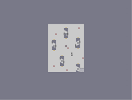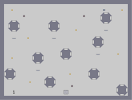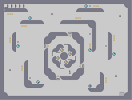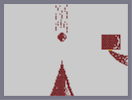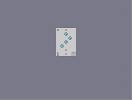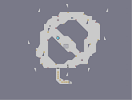mini steps bigger o da noes to the tip funmap3 the crack

Pages: (0)

@DemonzLunchBreak

I know, it was a joke. ; )

5/5

I'm cool...
0ED, 0ED, 0ED!

OK

WHO SIPED!!!!!!!!!!!!!!!!!!!!!!!!!!!!!!!!!!!!!!!!!!!!!!!!!!!!!!!!!!!!!!!!!!!!!!!!!!!!!!!!!!!!!!!!!!!!!!!!!!!!!!!!!!!!!!!!!!!!!!!!!!!!!!!!!!!!!!!!!!!!!!!!!!!!!!!!!!!!!!!!!!!!!!!!!!!!!!!!!!!!!!!!!!!!!!!!!!!!!!!!!!!!!!!!!!!!!!!!!!!!!!!!!!!!!!!!!!!!!!

indeed 4.5/5

omg

Cool!!! But its not better than Afternoon Dragon. 5/5

Probably not, Kirbs

but this is pretty good looking

A ded!? :O
4.5/5